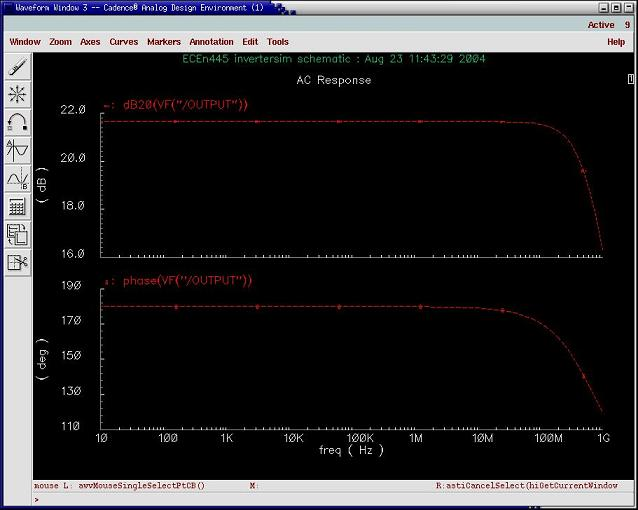Analog Tutorial 2: Simulating an Inverter

# Analog Tutorial 2: Simulating an Inverter

Overview

## Overview

The main goal of this tutorial is to walk through some of the different simulations using the analog simulator. Specifically, Transient, DC, and AC simulations will be covered. This tutorial will use the same inverter that was built in the previous tutorial. For this tutorial, the library name "ECEn445" is only an example. Your library can be named anything.

## Transient Simulation

Before doing a transient simulation, a new schematic needs to be set up that will source the inverter.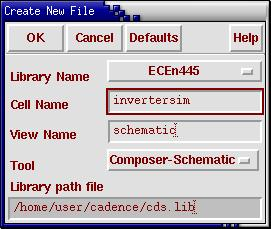From your cadence directory, start the Cadence tools by typing "icfb &". In your library, create a new cell labeled "invertersim", with a schematic view.

Once in the Virtuoso Schematic Editing window, select the "Instance" icon or type 'i' to place symbols on the schematic. Next, find the inverter you created in your library, and place it on the schematic. If you did not go through Tutorial 1: Building an Inverter, then you can find the symbol for the same inverter in the Tutorial_Components library.

Both found in the "NCSU_Analog_Parts" library, under "Voltage_Sources", place the vdc and vpulse components on the schematic. Connect vdc (DC Voltage Source) to vdd and vpulse (Pulse Voltage Source - Square Wave) to the input of the inverter, using the place wire function by typing 'w'. Also, create an output pin by typing 'p' and attach it to the output of the inverter and call it "OUTPUT". Don't forget to attach your grounds from the "Supply_Nets" category. Between using different functions such as the wire or pin placement, be sure to type 'ESC' to exit the previous function you were using. You will know if you have exited the function when the text (this text states the function being used) displayed at the bottom of the Virtuoso Schematic Editing window has disappeared.

When everything is attached, your schematic should look something like the picture below.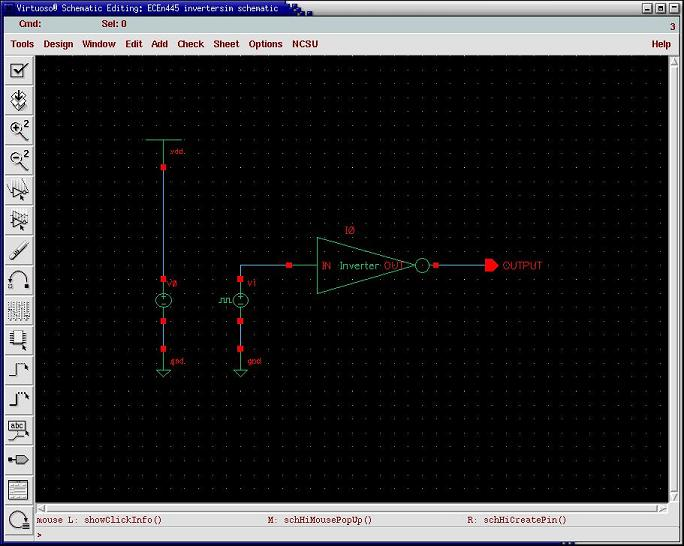Change the properties (type 'q') of the DC voltage source so that it has a 5 in the "DC voltage" parameter. Change the properties of the Pulse voltage source so that it has:

• Voltage 1 = 0V
• Voltage 2 = 5V
• Delay time = 0ns
• Rise time = 2ns
• Fall time = 2ns
• Pulse width = 25ns
• Period = 50ns
Remember, that you do not need to put the units ('V' or 's') because they will be entered in by default.

Check and save the schematic. Select Tools -> Analog Environment. The Cadence Analog Design Environment window should appear.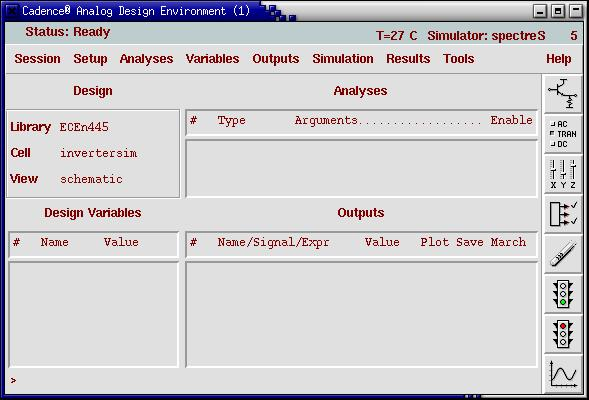The simulator in the upper right-hand corner should read "spectreS". We want to use spectreS in this case because the nmos and pmos models were written for spectreS. In other cases though, we will want to choose another simulator. This can be done by selecting Setup -> Simulator/Director/Host ... If your simulator does not read "spectreS", then change it.

Next, select Analyses -> Choose ... Select the "tran" analysis if it isn't already selected and enter 500n (500 nanoseconds) in the Stop Time. Make sure the box for Enabled is selected. Select OK.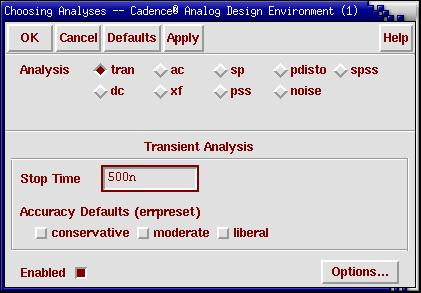****************************

Go to Setup -> Model Libraries

****************************

After you have setup the transient analysis, your Cadence Analog Design Environment window should look like the picture below: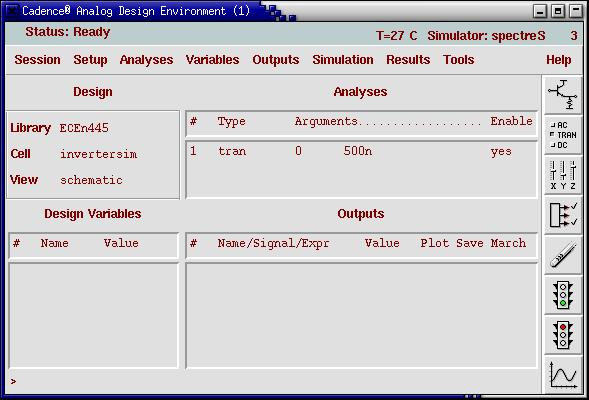Select the icon with the green traffic light or select Simulation -> Run. Verify that the simulation has completed successfully by checking the CIW. If any errors are present, scroll up the CIW to find them.

To view the input and output waveforms, select Results -> Direct Plot -> Transient Signal. Then, you will be directed to the Virtuoso Schematic Editing window and be prompted to select the signals that you want to display. Select the input and output signals of the inverter(make sure they are highlighted with a dashed line) to plot. Then, hit 'ESC'.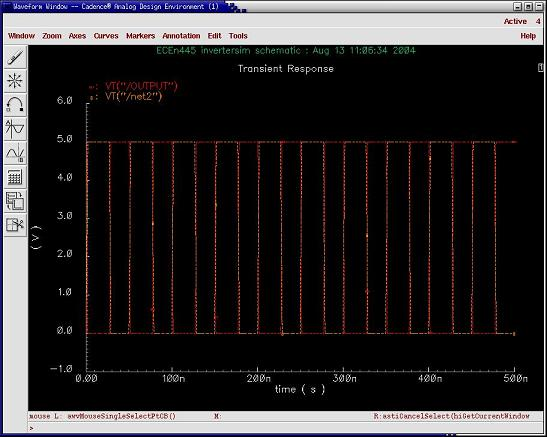The Waveform Window should appear with the plots overlaying eachother. Select the second to last icon labeled "Switch Axis Mode". This will put the waveforms on different plots.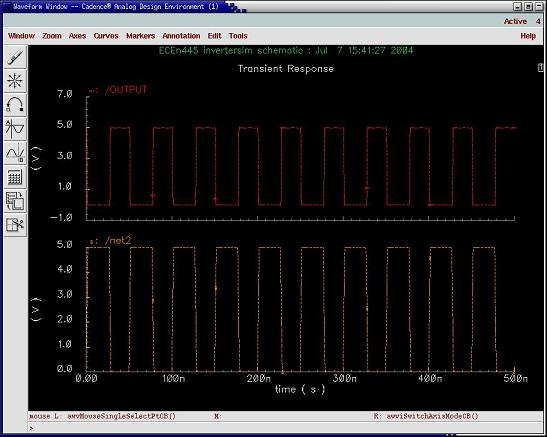Another approach to running the simulation and viewing the waveforms is to first select the signals to view and then run the simulation. This can be done by going to the Cadence Analog Design Environment window and selecting Outputs -> To Be Plotted -> Select On Schematic. After selecting this option, you will be brought to the Virtuoso Schematic Editing window. Select the input of the inverter and the output of the inverter. Make sure the nodes you have selected are highlighted. Type 'ESC' to exit the waveform select function. Now, go back to Cadence Analog Design Environment window and verify that your window looks like the picture below. Then, run the simulation by selecting the green light icon or by selecting Simulation -> Run. The Waveform Window will appear if it is not already open with the signals that you selected plotted.

## Getting Used to the Waveform Window

Now that you have plotted your waveforms, you need to be familiarized with the tools used to analyze them. One tool is the Waveform Calculator, but that will be discussed in the next section. Some basic hotkeys and options that you should be aware of are:
• z - Zoom in (after typing 'z', click the mouse to form the box that you want to zoom in)
• Z - Zoom out (after typing 'Z', click the mouse to form the box that you want to zoom out)
• ] - Fast zoom in
• [ - Fast zoom out
• F - Zoom to fit to the complete waveform
• Select Axes -> Options... - To turn the grid lines on/off and change other axis properties.
• b - Place crosshair marker B

## Using the Waveform Calculator

Now that you have completed a transient simulation of the inverter, you want to know the characteristics of the output, such as the rise time, the fall time, and the delay time. All of these characteristics, plus many more, can be calculated using the Waveform Calculator. In the Waveform Window, select the third to last icon that looks like a calculator, labeled "Calculator".The functions delay() and riseTime() can be use to calculate the delay time, and rise and fall times, respectively. These functions can be selected using the "Special Functions" menu or can be typed in manually. This tutorial will show you how to do it both ways. Knowing how to use the functions manually will enable the user to write the functions in a report without having to use the calculator. Besides what this tutorial teaches you, don't be afraid to explore the calculator more and find out what else it can do.

### riseTime

The riseTime function has 7 input arguments, but you only really need to worry about three of them. The rise time is defined as the time between 10% and 90% of the final value of the rising edge. Fall time is the same thing, but for the falling edge.

riseTime(voltage_waveform start_time t end_time t 10 90)

voltage_waveform - This argument is the actual waveform you want to find the rise time of. Use the VT function to indicate you are looking at the voltage waveform. Example: VT( "/OUTPUT" ) ...where /OUTPUT is the name of the waveform in the Waveform Window.

start_time - This argument can be represented by a number or a marker. A marker is probably easier to use. When calculating the rise time, this argument must occur before the rising of the waveform begins. When calculating the fall time, this argument must occur before the falling of the waveform begins.

end_time - This argument can be represented by a number or a marker. A marker is probably easier to use. When calculating the rise time, this argument must occur after the rising of the waveform begins. When calculating the fall time, this argument must occur after the falling of the waveform begins.

### delay

The delay function has 8 input arguments. The user has to indicate the name, voltage threshold, edge, and edge type of each waveform. This function calculates the timing between the voltage threshold of the rising edge of one waveform and the voltage threshold of the falling edge of another waveform. In most cases, the voltage thresholds will be set to 50% of their respective maximum voltage.

delay(voltage_waveform1,voltage_threshold1,edge_number1,"edge_type",voltage_waveform2,voltage_threshold2, edge_number2,"edge_type")

voltage_waveform1/2 - This argument is the actual waveform you want to find the rise time of. Use the VT function to indicate you are looking at the voltage waveform. Example: VT( "/OUTPUT" ) ...where /OUTPUT is the name of the waveform in the Waveform Window.

voltage_threshold1/2 - This argument requires a voltage threshold value to indicate either where the timing starts or ends.

edge_number1/2 - This argument indicates the n-th edge. So, if the argument was 2, then it would find the 2nd edge.

edge_type - This argument indicates the type of edge: rising, falling, or either.

### Placing Markers

Before calculating these values, let's define 2 vertical markers for the riseTime function. First, go to the Waveform Window and zoom in by typing 'z' and clicking your mouse to outline one of the transition areas of the waveforms (a rising edge of the output). Once you have zoomed in to the desired area, select Markers -> Vertical Marker ...Once the Vertical Markers window opens, select "Add Graphically" and in the Waveform Window, place the markers before and after the rising of the output begins and ends.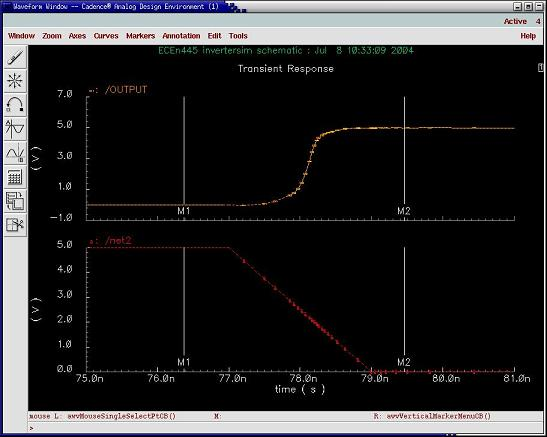### Calculating the Rise Time

#### Manually

Return to the Calculator window and type the following in the window:

riseTime(VT("/OUTPUT") M1 t M2 t 10 90)

After you have typed this command (without commas between arguments), select the enter button and the command should be replaced with a value. This value is your rise time.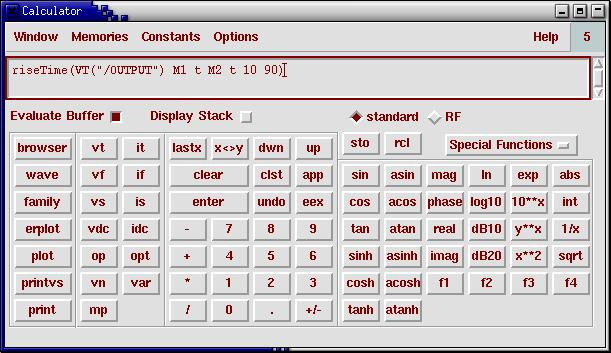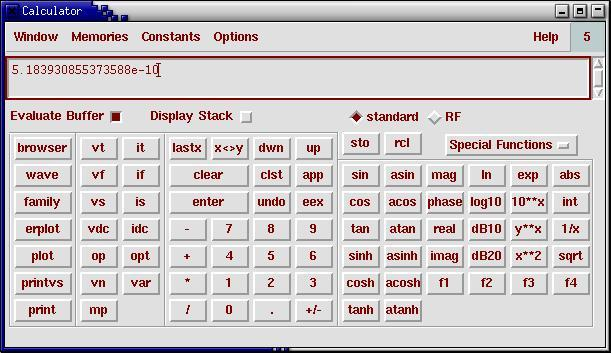#### Using the Calculator Buttons

Make sure that "Evaluate Buffer" is not selected. Click on the wave button, and then select the output waveform. Then, in the Calculator window, select "Special Functions" and then "riseTime" from the list. A new window entitled "Rise Time" will appear and you will prompted to enter initial and final values. Verify that the "y to x" buttons are selected, and type M1 and M2, respectively.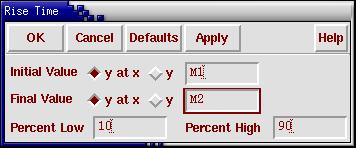Then, select OK and "Evaluate Buffer" in the Calculator window. The results will be displayed in the window.

### Calculating the Fall Time

Ensure that "Evaluate Buffer" is not selected. In the Waveform Window, type "F" to zoom out and fit the waveforms to the window. Place the markers (in the same order) around a falling edge of the output. Zoom in if you need to. Then repeat the steps above either manually or using the calculator buttons.

### Calculating the Delay

#### Manually

You do not need to use the markers for calculating the delay. Enter the following command (with arguments having commas between them) in the calculator window

delay(VT("/net2"),2.5,1,"either",VT("/OUTPUT"),2.5, 1,"either")

After selecting the enter button, the screen will tell you your delay time. If

#### Using the Calculator Buttons

First, ensure that "Evaluate Buffer" is not selected. Then, select the wave button and select the input waveform in the Waveform Window. Then, select "Special Functions" and "delay" from the list. A new window entitled "Threshold Delay" will appear. You can leave the default values. The calculator will have automatically placed the correct arguments in for the input and output waveforms.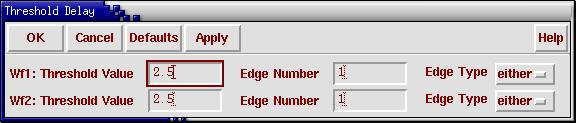Then, select OK and "Evaluate Buffer" in the Calculator Window. The calculator will have automatically placed the correct arguments in for the input and output waveforms. The results will be displayed in the window.

If you would like to test the accuracy of these tools, manually measure the rise, fall, and delay times and see how well they match up. It may be difficult to manually measure a timing as accurate as the functions can compute them though. A good method of calculating these values is using the crosshair markers.

### Using the Crosshair Markers

To calculate the rise time of the output, first zoom into a rising edge of the output in the Waveform Window.Then, type 'a' for the a-crosshair marker and place it (by viewing the x/y coordinates in the upper-left corner) at y = 0.5 (10% of final value). Then, type 'b' for the b-crosshair marker and place it at y = 4.5 (90% of final value). You may not be able to place the markers at these exact locations.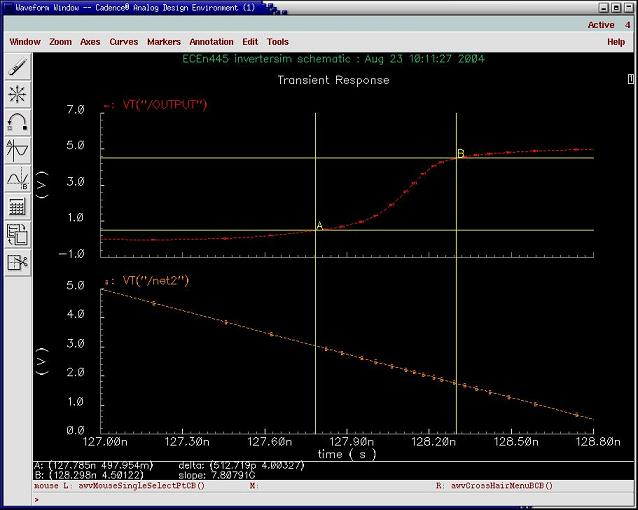Notice at the bottom left corner of the Waveform Window, that the crosshair markers have been listed, along with a delta value (x-coordinate of b minus x-coordinate of a).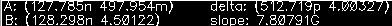Therefore, the rise time (delta value) is approximately 512.719 picoseconds. Remember, the calculator is probably more accurate because it is calculating the rise time between points at 0.5 V and 4.5 V. With the crosshair markers, for this example, the rise time was calculated for points 497.954 mV and 4.50122 V. You might want to try calculating the fall time and delay time using the crosshair markers and then compare these values to the calculator values.

## DC Simulation

For the DC simulation, we will sweep the input of the inverter, 0 to 5 volts. To do this, first open up the schematic that is set up to simulate the inverter. Then, select Tools -> Analog Environment, to open the Cadence Analog Design Environment window.

Verify that the simulator that you are using is "spectreS". If not, change the simulator to spectreS by selecting Setup -> Simulator/Directory/Host ... Now, select Analyses -> Choose ...

Select the DC box under the analysis list. Also, select "Component Parameter" and "Select Component". In the Virtuoso schematic window, select the voltage source that is connected to the input of the inverter. A list of parameters of the voltage source will appear. Choose "dc" and make sure in the analysis setup window, that the Sweep Range has "Start-Stop" selected and 0 and 5 are in the "Start" and "Stop" parameters, respectively. Also, make sure that the Sweep Type reads "Automatic".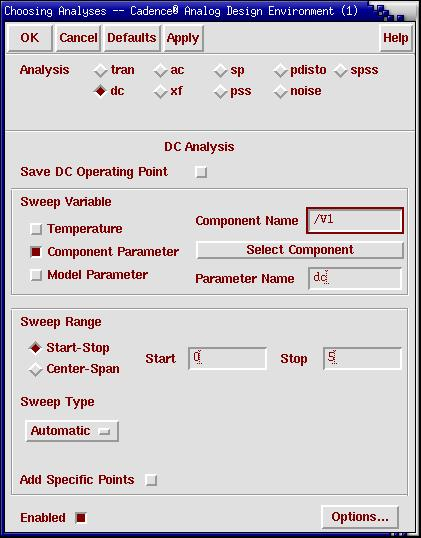Now, you are ready to simulate. Select the output and input of the inverter to plot and run the simulation. The DC Response should look something like the waveform below.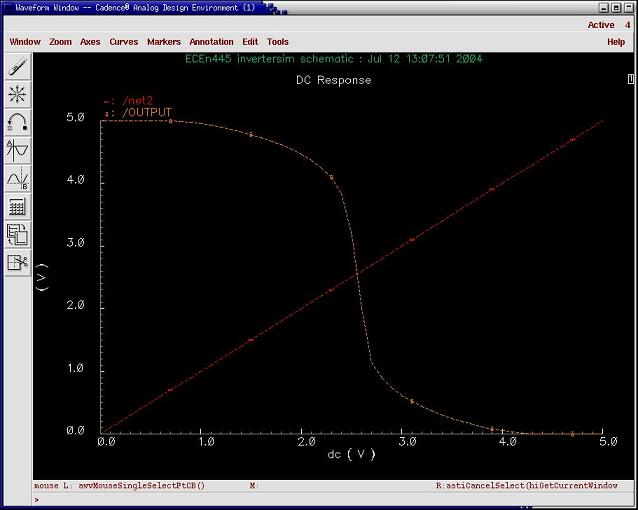Notice how the input and output cross in the linear (transition) region of the inverter. We will want to calculate this crossing value so that it can be used as a bias to the input of the inverter for future AC simulation. This can be accomplished either by eyeing it or more effectively by using the calculator.

Open the calculator by selecting the calculator icon. Make sure that "Evaluate Buffer" is unchecked. Select wave, and then click on the output waveform in the Waveform Window. Now, select "Special Functions" and choose "cross" in the Calculator window. When the "Threshold Crossing" window appears, select OK for the default values. Select "Evaulate Buffer" in the Calculator Window, and the cross value will be given. This should be roughly vdd/2 (vdd/2 is for an ideal inverter). Round off to the nearest hundreth and remember this value for AC simulation.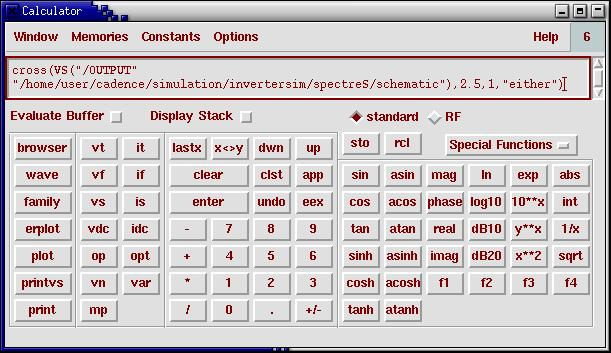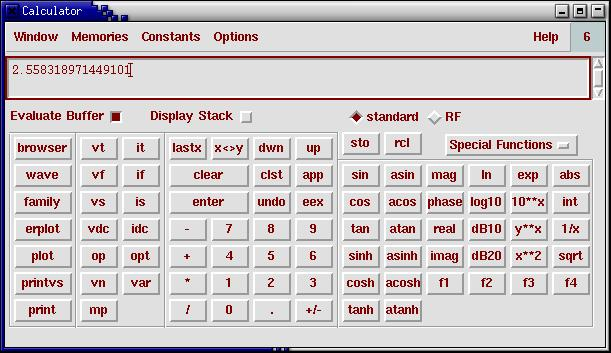## AC Simulation

Before doing an AC simulation, a change needs to be made to the schematic that is testing the inverter. The pulse source needs to be changed to a sine wave source. This source can found in the "NCSU_Analog_Parts" library, under the "Voltage_Sources" category. Change the following parameters:
1. AC magnitude = 1 V
2. AC phase = 0
3. Offset Voltage = 2.56 V (this value is what we computed from the DC simulation)
Check and save your schematic and choose Tools -> Analog Environment if you don't already have the Cadence Analog Design Environment window open. Select Analyses -> Choose ... When the Choosing Analyses window opens, select AC analysis. Make sure that "Frequency" is selected and 10 and 1000M are the start and stop frequencies, respectively. Also, select a logarithmic sweep with 10 points per decade. Choose OK.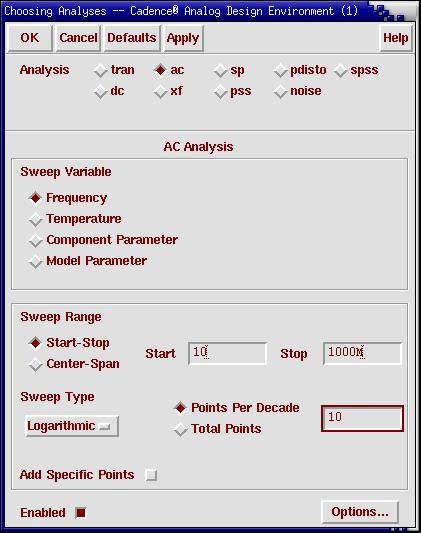Next, run the simulation. Select Results -> Direct Plot -> AC Magnitude & Phase in the Cadence Analog Design Environment window to view the frequency response of the AC simulation.